# Introduction to streamDepletr

## Modeling streamflow depletion

Streamflow depletion, defined as a reduction in streamflow resulting from groundwater pumping (Barlow et al., 2018), cannot be measured directly and therefore is always a modeled quantity. There are two general classes of groundwater models used to quantify streamflow depletion: analytical and numerical models. streamDepletr is a collection of analytical streamflow depletion models and related functions intended to make analytical streamflow depletion models more accessible.

However, anyone using analytical models should be aware that they have many more assumptions than numerical models, including:

• Homogeneous and isotropic aquifer.
• Constant transmissivity - aquifer is either confined, or unconfined with negligible head change due to pumping.
• Constant stream stage - head in stream does not lower or dry up due to pumping.
• Recharge does not change due to pumping.
• Negligible vertical groundwater flow, AKA the Dupuit-Forchheimer assumption holds.
• No streambank storage.
• Streams are linear and infinite in extent
• Aquifers are infinite in extent

These assumptions notwithstanding, analytical streamflow depletion models are useful tools for estimating groundwater pumping impacts on streamflow, in particular in settings where the time, data, or resources do not exist to create numerical models.

If you are interested in numerical models, I recommend you check out the excellent FloPy package for Python.

## Estimating capture fraction

streamDepletr has a variety of streamflow depletion models; two of the most commonly used are glover (Glover & Balmer, 1954) and hunt (Hunt, 1999). They differ in the the representation of the stream-aquifer interface: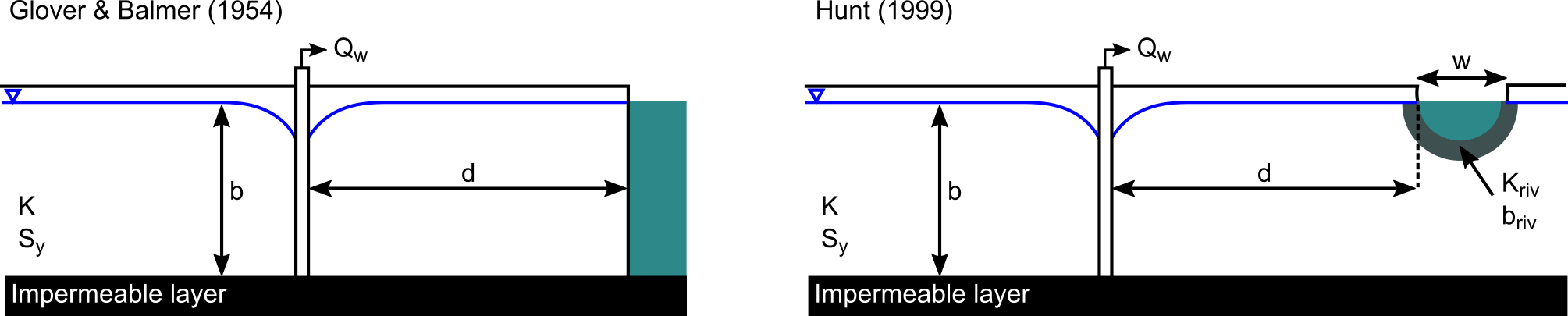glover is simpler and assumes a stream that fully penetrates the aquifer and no streambed resistance to flow. In contrast, hunt assumes the stream partially penetrates the aquifer and has a partially clogging streambed. hantush is rarely used but has intermediate functionality between glover and hunt and is included in the package for completeness.

To see how these compare, let’s consider a well 150 m from a stream in a 50 m thick, unconfined aquifer with a specific yield of 0.1 and a hydraulic conductivity of 1e-5 meters/second. For the hunt model we also need some information about the stream; we’ll say it’s width is 5 m, riverbed is 10% as conductive as the aquifer, and riverbed thickness is 1 m.

First, we’ll define the aquifer parameters common to both models:

times <- seq(1, 100) # time [days]
K <- 1e-5 * 86400 # hydraulic conductivity [m/d]
b <- 50 # aquifer thickness [m]
trans <- b * K # transmissivity [m2/d]
d <- 250 # well to stream distance [m]
Sy <- 0.1 # specific yield [-]

For hunt, we also need some information about flow properties of the streambed. We can estimate that using the streambed_conductance function:

str_cond <- streambed_conductance(
w = 5, # river width [m]
Kriv = 0.1 * K, # streambed K is 10% that of the aquifer
briv = 1
) # thickness of streambed

Now, we can use our analytical models to calculate the capture fraction (Qf), which is streamflow depletion expressed as a fraction of the pumping rate:

df_depletion <-
data.frame(
times = times,
Qf_glover = glover(t = times, d = d, S = Sy, Tr = trans),
Qf_hunt = hunt(t = times, d = d, S = Sy, Tr = trans, lmda = str_cond)
)

df_depletion |>
tidyr::pivot_longer(-times, values_to = "Qf", names_to = "model") |>
ggplot2::ggplot(aes(x = times, y = Qf, color = model)) +
geom_line() +
scale_y_continuous(limits = c(0, 1))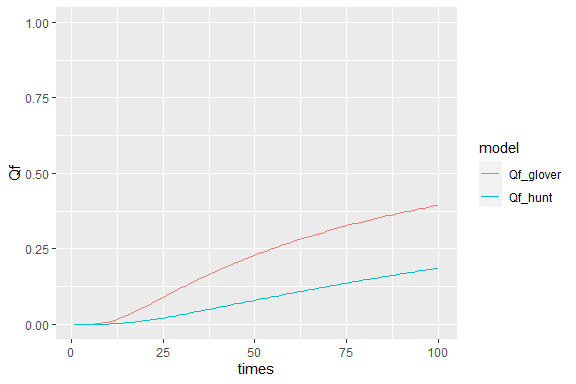To demonstrate the importance of the parameterization of the streambed in the hunt model, we can compare capture fraction at the end of the 100 day period:

df_depletion[dim(df_depletion), ] # glover is ~2x hunt
#>     times Qf_glover   Qf_hunt
#> 100   100 0.3950376 0.1860926

## Converting capture fraction to streamflow depletion

To convert capture fraction, Qf, to volumetric streamflow depletion, Qs, we simply multiply Qf by the pumping rate, Qw.

Qw <- 500 # pumping rate, [m3/d]
df_depletion$Qs_glover <- df_depletion$Qf_glover * Qw # streamflow depletion, [m3/d]
df_depletion$Qs_hunt <- df_depletion$Qf_hunt * Qw # streamflow depletion, [m3/d]

# plot results
df_depletion |>
dplyr::select(c("times", "Qs_glover", "Qs_hunt")) |>
tidyr::pivot_longer(-times, values_to = "Qs", names_to = "model") |>
ggplot2::ggplot(aes(x = times, y = Qs, color = model)) +
geom_line()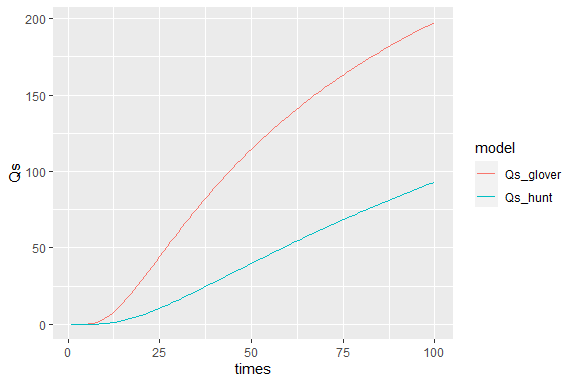## Intermittent pumping schedules

While glover and hunt were originally developed and described for continuous pumping, Jenkins (1968) demonstrated that the principles of superposition can be used to estimate depletion under intermittent pumping schedules. Let’s see what happens if we turn a well on/off 3 times during a two year period:

# define pumping schedule
t_starts <- c(10, 200, 400) # days that well turns on
t_stops <- c(60, 350, 700) # days that well turns off

# calculate depletion through time
df_intermittent <-
data.frame(
times = seq(1, 730),
Qs_intermittent =
intermittent_pumping(
t = seq(1, 730), starts = t_starts, stops = t_stops,
rates = rep(Qw, length(t_starts)),
method = "glover", d = d, S = Sy, Tr = trans
)
)

# plot - times when the well is turned on are shaded red
ggplot2::ggplot(
df_intermittent,
aes(x = times, y = Qs_intermittent)
) +
annotate("rect",
xmin = t_starts, xmax = t_stops,
ymin = -Inf, ymax = Inf, fill = "red", alpha = 0.5
) +
annotate("rect",
xmin = t_starts, xmax = t_stops,
ymin = -Inf, ymax = Inf, fill = "red", alpha = 0.5
) +
annotate("rect",
xmin = t_starts, xmax = t_stops,
ymin = -Inf, ymax = Inf, fill = "red", alpha = 0.5
) +
geom_line()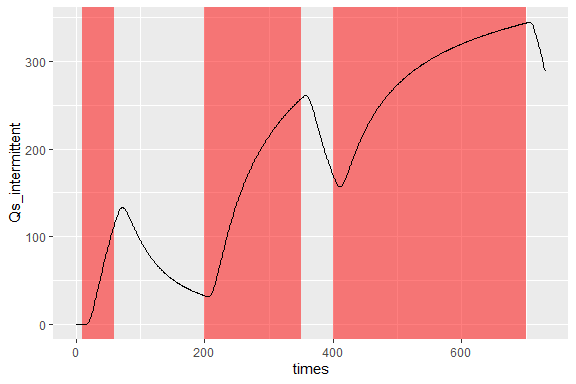We can also pump at different rates at different times; let’s see how that changes the estimated depletion:

pump_rates <- c(100, 1000, 100) # [m3/d] - must be same length as t_starts and t_stops
df_intermittent$Qs_variableRate <- intermittent_pumping( t = seq(1, 730), starts = t_starts, stops = t_stops, rates = pump_rates, method = "glover", d = d, S = Sy, Tr = trans ) # plot - times when the well is turned on are shaded red df_intermittent |> tidyr::pivot_longer(-times, values_to = "Qs", names_to = "pumpSchedule") |> ggplot2::ggplot(aes(x = times, y = Qs, linetype = pumpSchedule)) + annotate("rect", xmin = t_starts, xmax = t_stops, ymin = -Inf, ymax = Inf, fill = "red", alpha = 0.5 ) + annotate("rect", xmin = t_starts, xmax = t_stops, ymin = -Inf, ymax = Inf, fill = "red", alpha = 0.5 ) + annotate("rect", xmin = t_starts, xmax = t_stops, ymin = -Inf, ymax = Inf, fill = "red", alpha = 0.5 ) + geom_line()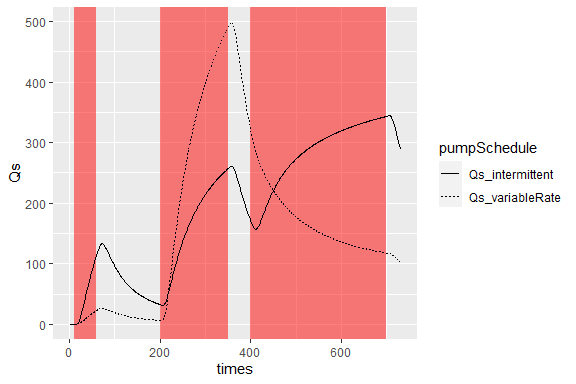## Working with real stream networks ### Preparing stream and well input data The most common ‘real world’ application for analytical models is estimating the impacts of a (proposed or existing) pumping well on a stream network. streamDepletr contains several functions to make this analysis as simple as possible. As an example, let’s consider the hypothetical case of a proposed high-capacity well in Wisconsin’s Sixmile Creek Watershed of Wisconsin. This watershed contains two US Geological Survey streamflow gauging stations, one on Sixmile Creek and one on Dorn Creek (a tributary). These gauging stations are both just upstream of the junction between Sixmile and Dorn creeks, providing us an opportunity to investigate how this proposed well would affect each of the two streams. Here is a map showing the scenario, as well as two water years of streamflow data from each gauging station: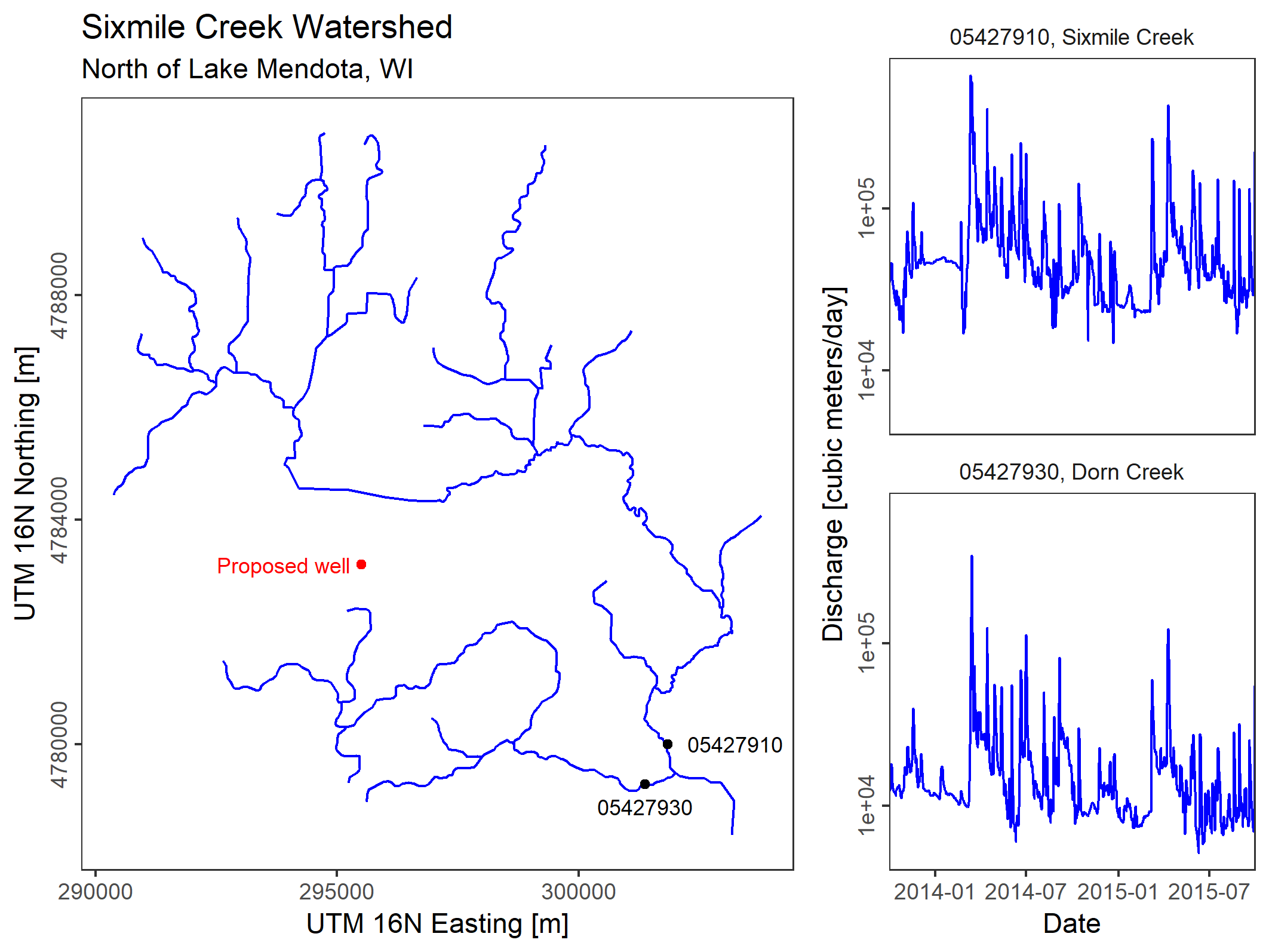The stream network and discharge data are included in the package (stream_lines and discharge_df, respectively). First, let’s define the properties of the well and the aquifer: # well properties Qw <- 1000 # well pumping rate [m3/d] wel_lon <- 295500 # easting of well [m] wel_lat <- 4783200 # northing of well [m] date_pump_start <- as.Date("2014-03-01") # pumping start date date_pump_stop <- as.Date("2015-08-01") # pumping stop date # aquifer properties K <- 1e-5 * 86400 # hydraulic conductivity [m/d] b <- 250 # aquifer thickness [m] trans <- b * K # transmissivity [m2/d] Sy <- 0.05 # specific yield [-] First, we need to determine the position of the well relative to the stream network. In streamDepletr this information is contained within the reach_dist_lat_lon data frame, which splits the stream network up into equally spaced points and determines the distance from each point to the well: rdll <- prep_reach_dist( wel_lon = wel_lon, wel_lat = wel_lat, stream_sf = stream_lines, reach_id = "reach", stream_pt_spacing = 5 ) head(rdll) #> reach dist lat lon #> 1 07090002008187 5253.566 4788325 296653.6 #> 2 07090002008187 5248.909 4788321 296650.8 #> 3 07090002008187 5244.252 4788317 296648.0 #> 4 07090002008187 5239.596 4788313 296645.2 #> 5 07090002008187 5234.941 4788309 296642.4 #> 6 07090002008187 5230.286 4788305 296639.6 Now, let’s figure out what would happen if we assumed all groundwater pumping depleted the closest stream reach to the well: # figure out which stream is closest closest_reach <- rdll[which.min(rdll$dist), "reach"]
closest_dist <- rdll[which.min(rdll$dist), "dist"] closest_stream <- stream_lines$stream[stream_lines$reach == closest_reach] closest_discharge <- subset(discharge_df, stream == closest_stream) # since time inputs for the streamflow depletion models are numeric (not dates), # we need to figure out the start and stop date in days since the start of our period of interest t_pump_start <- as.numeric(date_pump_start - min(closest_discharge$date))
t_pump_stop <- as.numeric(date_pump_stop - min(closest_discharge$date)) times <- as.numeric(closest_discharge$date - min(closest_discharge$date)) # calculate depletion - since the pumping starts and stops during our period of interest, # we will use the intermittent_pumping function even though it is only one pumping cycle Qs <- intermittent_pumping( t = times, starts = t_pump_start, stops = t_pump_stop, rates = Qw, method = "glover", d = closest_dist, S = Sy, Tr = trans ) # plot capture fraction through time - the shaded interval indicates when pumping is occurring data.frame(date = closest_discharge$date, Qs = Qs) |>
ggplot2::ggplot(aes(x = date, y = Qs)) +
annotate("rect",
xmin = date_pump_start, xmax = date_pump_stop,
ymin = -Inf, ymax = Inf, fill = "red", alpha = 0.5
) +
geom_line() +
scale_y_continuous(name = "Qs, Streamflow Depletion [m3/d]")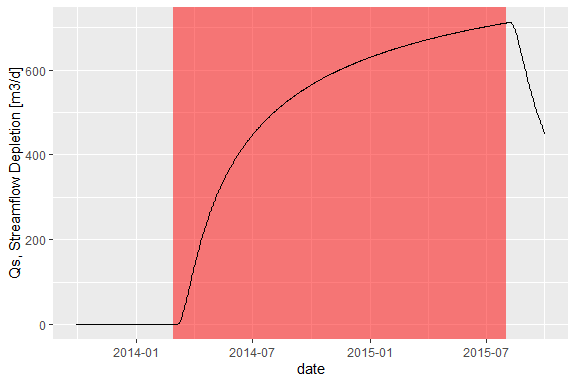If we assume that there are no changes to surface water-groundwater exchange elsewhere in the network, we can estimate the streamflow at the gauging station as the discharge in the no-pumping scenario minus the streamflow depletion.

# calculate streamflow
closest_discharge$Q_pumped <- closest_discharge$Q_m3d - Qs

closest_discharge |>
tidyr::pivot_longer(cols = c("Q_m3d", "Q_pumped"), names_to = "variable", values_to = "discharge_m3d") |>
ggplot2::ggplot(aes(x = date, y = discharge_m3d, color = variable)) +
annotate("rect",
xmin = date_pump_start, xmax = date_pump_stop,
ymin = -Inf, ymax = Inf, fill = "red", alpha = 0.5
) +
geom_line() +
coord_trans(y = scales::log1p_trans())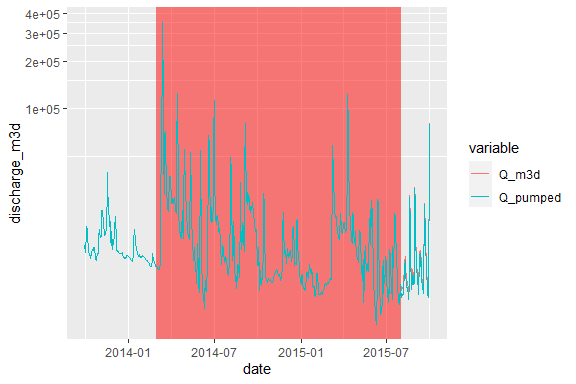### Integrating analytical models with depletion apportionment equations

In the example above, we assumed that all depletion occurred from the stream reach closest to the proposed well. This is a common approach, as one of the assumptions for most analytical models is that there is only one stream with a perpendicular aquifer of infinite extent. The real world, of course, is made up of many nonlinear streams. To deal with real stream networks, streamDepletr includes a variety of depletion apportionment equations which distribute the depletion calculated using the analytical models to different reaches within a stream network. These depletion apportionment equations are described in Zipper et al. (2018) and shown here (modified from Zipper et al., Figure 1):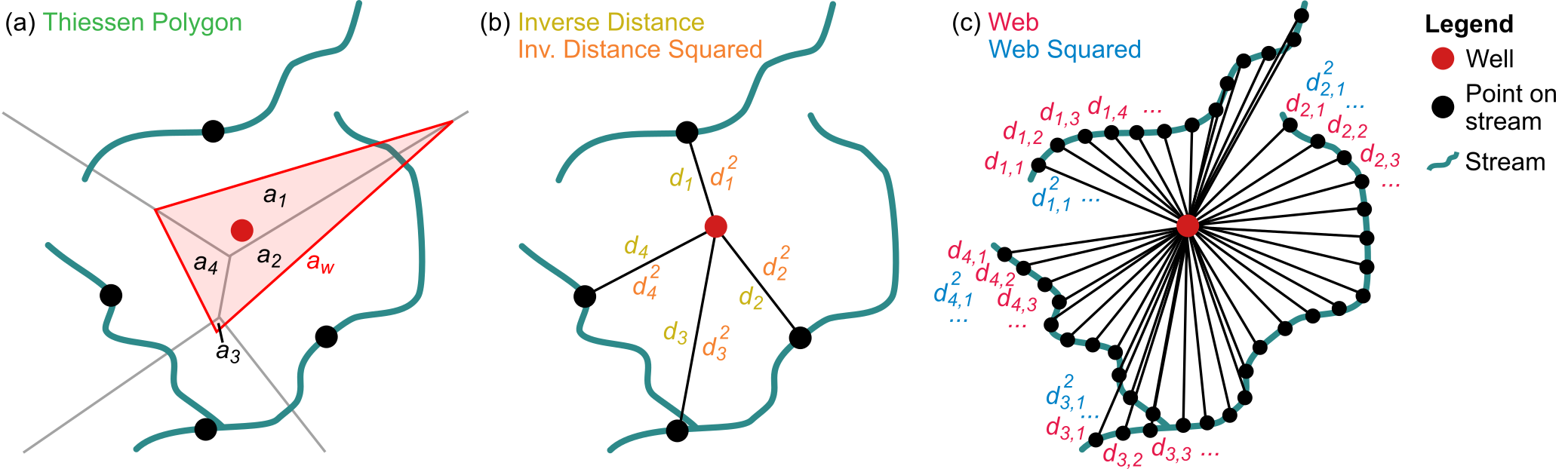Briefly:

• The Thiessen polygon method (apportion_polygon) uses the point on each stream reach closest to the well of interest to create Thiessen (or Voronoi) polygons including and ignoring the well, and weights streamflow depletion based on the area of overlap between the polygon associated with each stream reach and the polygon associated with the well.
• The inverse distance and inverse distance squared methods (apportion_inverse) also use the point on each stream closest to the well of interest, but weight depletion based on the distance between the well and each stream reach ; relative to the linear method, the squared method gives more weight to the closer stream reaches.
• The web and web squared methods (apportion_web) use the same inverse distance approach but divide each stream reach into a series of evenly spaced points to explicitly include stream geometry, instead of only using the closest point on each reach.

Zipper et al. (2018) found that apportion_web with a weighting factor (w) of 2 provided the best match with more complex, process-based streamflow depletion models.

The appropriate procedure to integrate the depletion apportionment equations and analytical models is:

1. Figure out how far away you want to distribute depletion
2. Calculate the fraction of depletion corresponding to each stream (frac_depletion) reach using the apportion_* functions.
3. Use the analytical models to estimate capture fraction (Qf’) occurring in each stream reach, ignoring all other reaches.
4. Multiply frac_depletion*Qf to estimate the depletion in each stream reach.

First, we’ll use the depletion_max_distance function to determine our the depletion apportionment radius as the area that will depleted by at least 1% of the pumping rate during the pumped interval:

max_dist <- depletion_max_distance(
Qf_thres = 0.01, method = "glover", d_max = 10000,
t = (t_pump_stop - t_pump_start), S = Sy, Tr = trans
)
max_dist
#>  5400

First, we’ll calculate depletion apportionment using the inverse distance squared method:

fi <- apportion_inverse(reach_dist = rdll, w = 2, max_dist = max_dist)
#>            reach frac_depletion
#> 1 07090002007664    0.008159978
#> 2 07090002007665    0.009153897
#> 3 07090002007666    0.010842769
#> 4 07090002007667    0.031301604
#> 5 07090002007668    0.026456416
#> 6 07090002007669    0.269197956

Let’s look at where depletion is occurring:

# merge fi with stream network shapefile
stream_lines_fi <- dplyr::left_join(stream_lines, fi, by = "reach")

# any NA values means they are outside the max_dist and should be set to 0
stream_lines_fi$frac_depletion[is.na(stream_lines_fi$frac_depletion)] <- 0

# cut frac_depletion into groups
stream_lines_fi$frac_depletion_intervals <- cut(stream_lines_fi$frac_depletion,
breaks = c(0, 0.05, 0.1, 0.2, 1),
labels = c("<5%", "5-10%", "10-20%", ">20%"),
include.lowest = T
)

# plot
ggplot2::ggplot(stream_lines_fi, aes(color = frac_depletion_intervals)) +
geom_sf() +
scale_color_manual(
name = "Fraction of Depletion", drop = F,
values = c("blue", "forestgreen", "orange", "red")
) +
theme_bw() +
theme(
axis.text.y = element_text(angle = 90),
panel.grid = element_blank(),
legend.position = "bottom"
)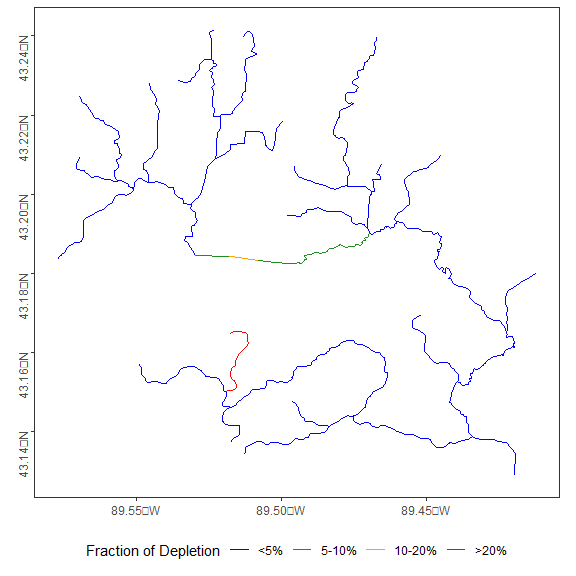Looks like some of the depletion is sources from Sixmile Creek after all - at least 10% within a single reach! We can use dplyr to determine the portion of depletion in Dorn and Sixmile creeks:

fi <-
dplyr::left_join(fi, unique(stream_lines[, c("reach", "stream")]), by = "reach")

fi |>
dplyr::group_by(stream) |>
dplyr::summarize(sum_depletion = sum(frac_depletion))
#> # A tibble: 2 × 2
#>   stream        sum_depletion
#>   <chr>                 <dbl>
#> 1 Dorn Creek            0.426
#> 2 Sixmile Creek         0.574

Wow- it turns out the well is capturing about the same amount of depletion from Sixmile and Dorn! This is likely because, while Dorn Creek is closer, Sixmile is more exposed to the well (the stream reach is oriented perpendicular to a line drawn between the well and the closest point on the stream).

Now, let’s calculate the analytical depletion timeseries for each reach. For the distance between the well and the stream (d), we’ll use the closest point on each reach:

fi <-
rdll |>
subset(reach %in% fi$reach) |> # only calculate for reaches with some depletion dplyr::group_by(reach) |> dplyr::summarize(dist_min = min(dist)) |> dplyr::left_join(fi, ., by = "reach") # join to data frame with apportionment head(fi) #> # A tibble: 6 × 5 #> reach dist_min frac_depletion stream geometry #> <chr> <dbl> <dbl> <chr> <LINESTRING [m]> #> 1 07090002007664 4488. 0.00816 Dorn Creek (298655.3 4780012, 298665 4… #> 2 07090002007665 4238. 0.00915 Dorn Creek (297990.4 4779772, 298005.8… #> 3 07090002007666 3894. 0.0108 Dorn Creek (295599.4 4778972, 295616.4… #> 4 07090002007667 2292. 0.0313 Dorn Creek (295196.5 4780306, 295344.7… #> 5 07090002007668 2493. 0.0265 Dorn Creek (295095.7 4780741, 295083.6… #> 6 07090002007669 781. 0.269 Dorn Creek (295207.7 4782372, 295233.6… We want to calculate the capture fraction for each stream reach (which has a unique distance) and at all timesteps. The simplest way to do this is by looping over each stream reach: for (r in 1:length(fi$reach)) {
df_r <- data.frame(
stream = fi$stream[r], reach = fi$reach[r],
frac_depletion = fi$frac_depletion[r], times = times, date = closest_discharge$date,
Qs_analytical =
intermittent_pumping(
t = times,
starts = t_pump_start,
stops = t_pump_stop,
rates = Qw,
method = "glover",
d = fi$dist_min[r], S = Sy, Tr = trans ) ) if (r == 1) { df_all <- df_r } else { df_all <- rbind(df_all, df_r) } } Now it is simple to calculate the estimated Qs considering apportionment equations: df_all$Qs_apportioned <- df_all$Qs_analytical * df_all$frac_depletion

Let’s look at the trajectory of each stream reach over time, with a different line for each stream reach:

ggplot2::ggplot(df_all, aes(x = date, y = Qs_apportioned, group = reach, linetype = stream)) +
annotate("rect",
xmin = date_pump_start, xmax = date_pump_stop,
ymin = -Inf, ymax = Inf, fill = "red", alpha = 0.5
) +
geom_line()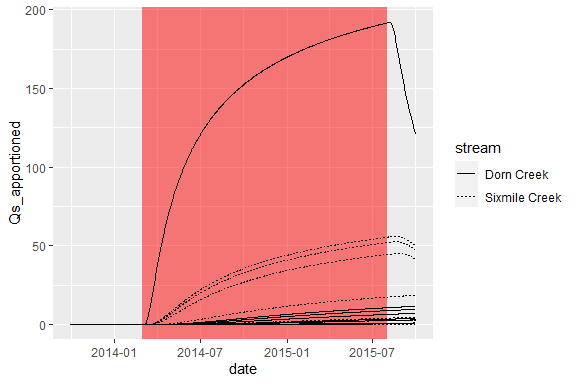Hmm… It doesn’t look like the Sixmile Creek lines would add up to equal the same amount as the Dorn Creek lines, but I thought we showed above that depletion would be 50/50? Not necessarily - what’s happening is that, while the depletion apportionment equations estimate an approximately equal apportionment (fi) for the two tributaries, but because the Sixmile Creek tributaries are further away the calculated streamflow depletion (Qs_analytical) is lower for Sixmile.

Maybe we want to know which stream reaches are most affected at the end of the pumping period:

df_all |>
subset(date == date_pump_stop & Qs_apportioned >= 20)
#>              stream           reach frac_depletion times       date
#> 4320     Dorn Creek  07090002007669     0.26919796   669 2015-08-01
#> 15270 Sixmile Creek  07090002007687     0.09663108   669 2015-08-01
#> 18190 Sixmile Creek 070900020081892     0.08634335   669 2015-08-01
#> 18920 Sixmile Creek 070900020081893     0.10130495   669 2015-08-01
#>       Qs_analytical Qs_apportioned
#> 4320       711.8453      191.62729
#> 15270      537.5491       51.94395
#> 18190      514.2598       44.40291
#> 18920      547.0854       55.42245

Finally, let’s take a look at streamflow for the two tributaries through time:

df_all |>
# sum depletion for all reaches in each tributary
dplyr::group_by(stream, date) |>
dplyr::summarize(Qs_sum = sum(Qs_apportioned)) |>
# join with raw discharge data
dplyr::left_join(discharge_df, by = c("date", "stream")) |>
# calculate depleted streamflow
transform(Q_depleted = Q_m3d - Qs_sum) |>
# melt for plot
tidyr::pivot_longer(
cols = -c("stream", "date", "Qs_sum"),
names_to = "variable",
values_to = "discharge_m3d"
) |>
# plot
ggplot2::ggplot(aes(x = date, y = discharge_m3d, color = variable)) +
annotate("rect",
xmin = date_pump_start, xmax = date_pump_stop,
ymin = -Inf, ymax = Inf, fill = "red", alpha = 0.5
) +
geom_line() +
facet_wrap(stream ~ ., ncol = 1) +
coord_trans(y = scales::log1p_trans())
#> summarise() has grouped output by 'stream'. You can override using the
#> .groups argument.`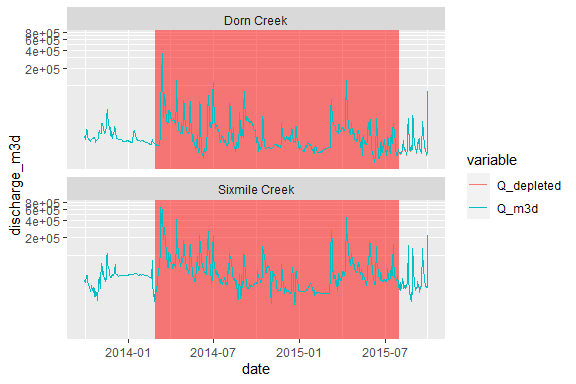In this case, the depletion from this well is fairly small relative to the overall discharge.